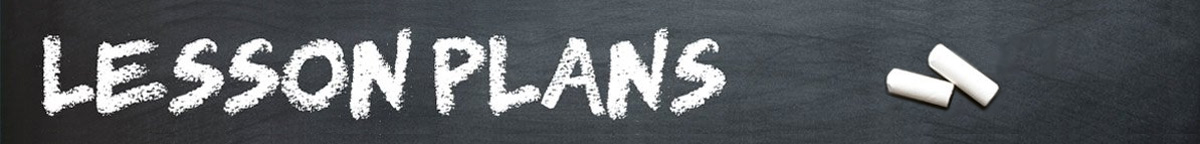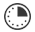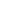# Ice Cream Cones, Baseballs, and Cans

JRDAssist
Middle School and High School Teacher

Students will work in pairs to practice finding the volume of cones, cylinders, and spheres using everyday objects.

##Length of Time: 1-2 Class Periods

Featured Programs:

### Common Core Alignment

CCSS.Math.Content.8.G.C.9 - Know the formulas for the volumes of cones, cylinders, and spheres and use them to solve real-world and mathematical problems.

## Objectives & Outcomes

Using everyday objects the students will be able to find the volume of cones, cylinders, and spheres using the formulas for each.  The students will be able to create problems for peers to solve related to the objects.

## Materials Needed

List of formulas to find the volume of a cone, sphere, and cylinder, calculators

*Students should be asked a week or two ahead of time to collect empty containers or objects such as cans, cones, different sizes of balls, ice cream scoops, lids, etc.  Anything that is sphere, cone, or cylinder-shaped.

Prepare ahead of time:  Have an example object such as an empty tennis ball container and tennis ball (or other objects) to explain instructions to students.  Sort collected objects to distribute to student pairs.

## Procedure

### Opening to Lesson

• The teacher will display sample objects to students
• Ask students what is similar between each object (circular shape included)
• Display the volume formulas for each shape

### Body of Lesson

#### Modeling

• Ask students what kind of question can be asked about the sample objects besides simply finding their volumes
• Allow students to respond (An example might be: How many golf balls may fit inside a tennis ball container?)
• Tell students they will be asked to use containers to measure and create word problems for peers

#### Guided Practice

• Pair students
• Distribute volume formula page to each student
• Distribute some collected objects to pairs of students (Number of objects will be based on the length of time lesson will be presented.)
• Tell students they are first to find the volume of each object
• Next, they are to create a predetermined number of word problems to be solved by their peers
• Encourage students to be creative. They can also use fractions of an object.  (For example, an ice cream scoop is half of a sphere, a question using a cone or cylinder could be:  How many scoops of ice cream will fit in...?)
• Students must also create the questions using correct grammar, punctuation, etc. There should also be an answer key and explanation for solving included.
• Once all students have completed the predetermined number of questions, the pairs of students will exchange them with other pairs of students to solve each other’s created word problems.
• Depending on the time frame for the lesson, the exchanging could occur more often.
• The teacher should monitor the work of the students as they create and solve the problems during class time.

#### Independent Practice

• For homework: Students will find two objects at home and create 3 to 5 word problems using the new objects.

### Closing

Allow students to share homework assignment, discuss the reasons it is important to accurately know the volume of objects.  (Use examples of gas tanks, propane containers, etc.)

## Assessment & Evaluation

Worksheet or other assessment tool with word problems to find the volume of cones, cylinders, and spheres.

## Modification & Differentiation

Students may work alone in or in larger groups; assign specific pairs to do only cylinders, spheres, or cones or combination; use created word problems on a test or quiz; do not allow the use of calculators.

Read more on teaching math.

## Related Lesson Plans

##### Order in the Math Class

The students will physically review the order of operations.

##### Interest-ing Math

Students will learn about simple interest and how to calculate the real cost of a loan, credit card, and other types of borrowing.

##### Prices and Percentages

Using a current list of prices for food and clothing, the students will practice math skills related to percentages.

##### Numbers By The Book

This lesson will allow students to show a relationship between numbers and a visual representation in a manner that can be used for younger students.

##